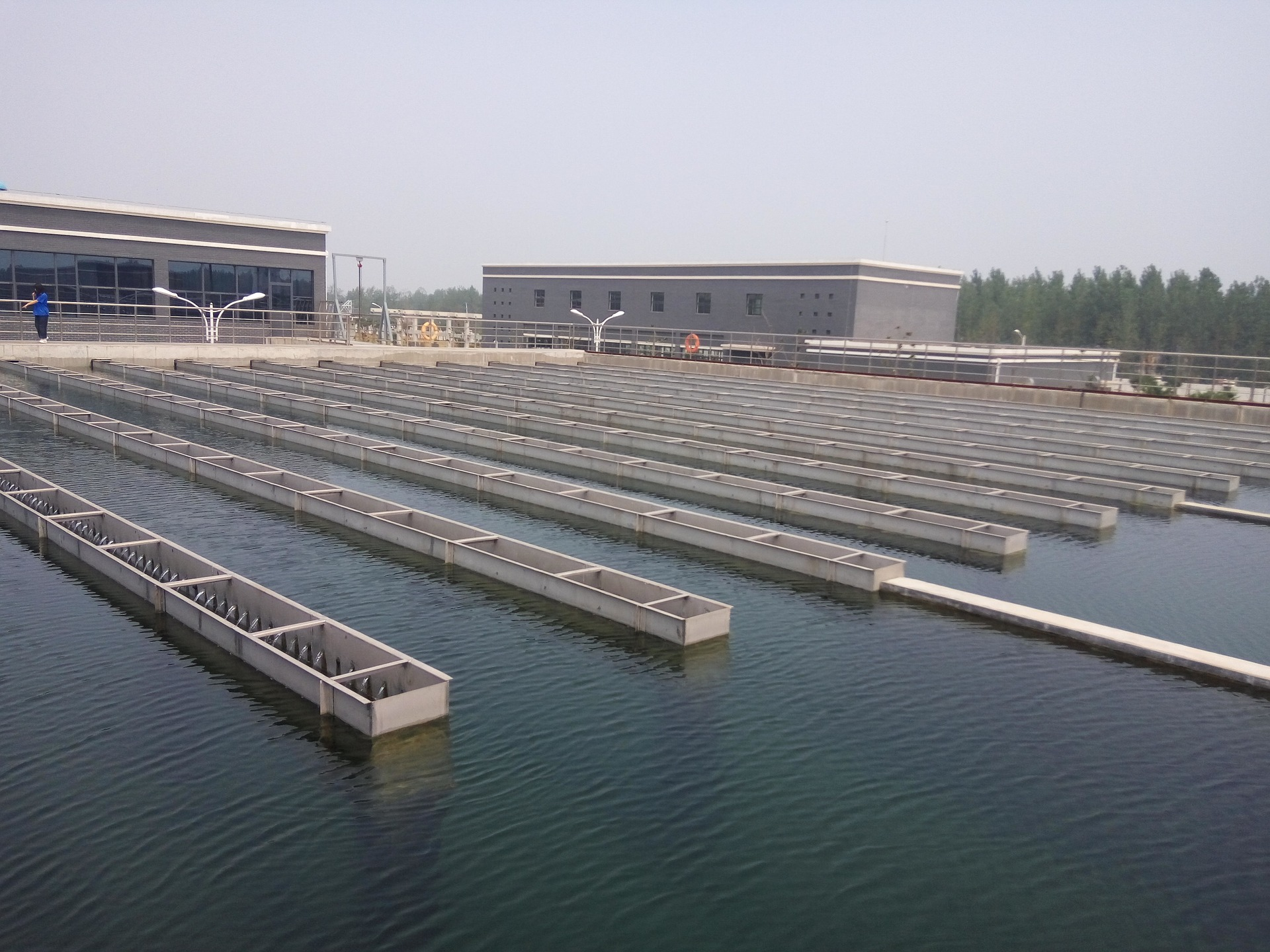# Water Treatment Plant Capacity Calculation

In this post, I want to share you how to calculate water treatment plant capacity. I used this term to avoid misunderstanding.

• Raw water is untreated water coming from water source, such as from wellwater, river, or seawater
• Utility water is raw water which has been treated and used in plant, such as for personal hygiene (flushing), utility station, cooling tower make up
• Potable water is sufficiently high quality water which is provided for drinking, cooking, laundry, and safety shower/eye wash
• Demineralized water is treated water which is usually used as chemical dilution of MDEA or boiler feed water

Water treatment plant capacity calculation begins with demin water demand, potable water demand, utility water demand, and the last is raw water demand.

Demineralized Water Demand

I don’t have experience in calculating demineralized water demand for amine contactor or chemical dilution. So, in this post, I assume demineralized water is used as boiler feed water. Let say we have boiler with capacity of 10 ton/hour. Assume blow down rate is 3%.

Demin water demand is = (1+3%) x 10 ton/hour = 10.3 ton/hour

Assuming density of water is 995 kg/m3 so, demin water demand is 10.35 m3/h or 45.57 USgpm.

Potable Water Demand

Let us assume potable water is provided for safety shower/eye washer and building.

1. Emergency shower = 20 USgpm (based on ANSI/ISEA Z358.1, operated for 15 minutes a day)
2. Eye wash = 3 USgpm (based on ANSI/ISEA Z358.1, operated for 15 minutes a day)
3. Drinking water user, assume number of person 50 person

Normal consumption includes drinking, cooking, and laundry

Drinking = 2 L/person/day

Cooking = 60 L/person/day

Laundry = 30 L/person/day

Total = 92 L/person/day. Let us take 100 L/person/day for this calculation

For 50 person, the total is = 5000 L/day or 0.92 USgpm

Peak demand is typically 5-10 L/person/h. So we take 10 L/person/h (total = 500 L/h or 2 USgpm)

Potable water demand must consider peak demand = 20 + 3 + 2.2 = 25.2 USgpm

Utility Water Demand

Let us assume uitlity water is provided for personal hygiene, utility connection, and cooling water make up.

1. Personal hygiene (including WC flushing)

Consumption = 110 L/person/day

Contingency = 11 L/person/day (10%)

Total = 121 L/person/day

Assuming 50 person, so total is 6050 L/day or 1.1 USgpm

1. Utility station

Consumption = 22 USgpm

1. Cooling water makeup

Cooling water make up is function of evaporation, drift loss, and blowdown.

To calculate cooling water makeup, let us assume:

Circulation flow = 1000 USgpm

Inlet temperature = 95 F

Outlet temperature = 85 F

Cycle of concentration = 5

Drift loss = 0.2% of circulation flow (varies between 0.1% and 0.2%)

Evaporation loss = 0.00085 x delta T x circulation flow = 0.00085 x (95-85) x 1000 = 8.5 USgpm

Drift loss = 0.2% x circulation flow = 0.2% x 1000 USgpm = 2 USgpm

Blowdown = evaporation loss/ (Cycle of concentration – 1) = 8.5/(5-1) = 2.13 USgpm

Cooling water makeup = evaporation loss + drift loss + blowdown = 8.5 + 2 + 2.13 = 12.63 USgpm

Utility water demand = 1.1 + 22 + 12.63 = 35.73 USgpm (peak)

After we identified all water demand, then put the demand in water balance.

From water balance above we can see that :

• Capacity of utility water package (using filtration & reverse osmosis) = 252.06 USgpm
• Capacity of potable water package = 25.2 USgpm
• Capacity of demineralized water package = 45.57 USgpm

The above capacity is not considering storage tank at each type of water. If you use store the water at respective storage tank (utility water storage tan, potable water storage tank, and demineralized water storage tank), you can reduce the capacity of each water treatment package. The storing days should be enough so that water requirement is always fulfilled. Take example, 3 days storing time. Water capacity can be reduced by 50%, so that:

• Capacity of utility water package = 126.03 USgpm
• Capacity of potable water package = 12.6 USgpm
• Capacity of demineralized water package = 22.78 USgpm
• Capacity of utility water distribution pump = 35.73 USgpm (based on previous calculation)
• Capacity of potable water distribution pump = 25.2 USgpm (based on previous calculation)
• Capacity of demineralized water distribution pump = 45.57 USgpm (based on previous calculation)

## One thought to “Water Treatment Plant Capacity Calculation”

1.dilipkapasi says:

For a small community of 200 homes, how do I calculate the size of water plant required in suburban USA using well water as source? My projected basis is 2 adults, 2 children in each of the 200 houses, 100 gpd usage per family x 120 [instead of 200 as not all use water at the same time]. Storage tank to provide easing of usage. 25-40 MGPD